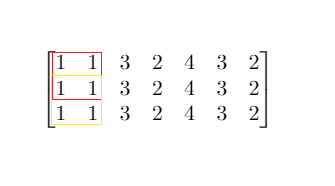## 704. 二分查找

• 你可以假设 nums 中的所有元素是不重复的
• n 将在 [1, 10000] 之间
• nums 的每个元素都将在 [-9999, 9999] 之间

## 153. 寻找旋转排序数组中的最小值

( 例如，数组 `[0,1,2,4,5,6,7]` 可能变为 `[4,5,6,7,0,1,2]` )。

UPDATE: 2020.7.22

## 154. 寻找旋转排序数组中的最小值 II

( 例如，数组 `[0,1,2,4,5,6,7]` 可能变为 `[4,5,6,7,0,1,2]` )。

• 这道题是 寻找旋转排序数组中的最小值 的延伸题目。
• 允许重复会影响算法的时间复杂度吗？会如何影响，为什么？

(UPDATE: 2020.7.22)

## 33. 搜索旋转排序数组

( 例如，数组 `[0,1,2,4,5,6,7]` 可能变为 `[4,5,6,7,0,1,2]` )。

1ms，99% 纯 if 判断** target mid 的位置，然后选择移动 lo 还是 hi**，一开始我随便找了几组数然后就开始写，写到后面发现都是 bug😂，这里画个图很方便`(nums[mid]>=nums)==(target>=nums)` 这一步很巧妙，满足这个关系就说明 mid 和 target 在同一段，不用变化，可以直接求，否则就根据 mid 的位置考虑如何变化

## 81. 搜索旋转排序数组 II

( 例如，数组 `[0,0,1,2,2,5,6]` 可能变为 `[2,5,6,0,0,1,2]` )。

• 这是 搜索旋转排序数组 的延伸题目，本题中的 `nums` 可能包含重复元素。
• 这会影响到程序的时间复杂度吗？会有怎样的影响，为什么？

WA 哭了，好难搞，要是在工程上我肯定直接遍历了，太细节了这波

（update：2020/4/8）和上面的搜索最小值一样，如果只是中间重复没有任何影响，但是如果是头尾重复，那么问题就大了，我们就没办法通过头或者尾的值，判断 target 和 mid 所在的区间，所以我们可以开始先对头尾去重，这样就可以方便判断 target 和 mid 所在区间，比如 1 0 1 1 1 就可以变成 1 0，然后再进行二分就很简单了

## 744. 寻找比目标字母大的最小字母

• 如果目标字母 `target = 'z'` 并且字符列表为 `letters = ['a', 'b']`，则答案返回 `'a'`

1. `letters`长度范围在`[2, 10000]`区间内。
2. `letters` 仅由小写字母组成，最少包含两个不同的字母。
3. 目标字母`target` 是一个小写字母。

## 34. 在排序数组中查找元素的第一个和最后一个位置

1ms ，99% 核心就是两次二分，分别向左和向后二分整个数组， 在相等的时候并不返回，多判断一下，左边的就控制 hi 向左边继续找，右边就控制 lo 向右边继续找，直到下一个不等于 target 就返回，和上面一题一样都是二分的变种

## 面试题 53 - II. 0～n-1 中缺失的数字

`1 <= 数组长度 <= 10000`

## 852. 山脉数组的峰顶索引

• A.length >= 3
• 存在 0 < i < A.length - 1 使得 A < A < … A[i-1] < A[i] > A[i+1] > … > A[A.length - 1]

## 1095. 山脉数组中查找目标值

（这是一个 交互式问题

• `A < A < ... A[i-1] < A[i]`
• `A[i] > A[i+1] > ... > A[A.length - 1]`

• `MountainArray.get(k)` - 会返回数组中索引为`k` 的元素（下标从 0 开始）
• `MountainArray.length()` - 会返回该数组的长度

`MountainArray.get` 发起超过 `100` 次调用的提交将被视为错误答案。此外，任何试图规避判题系统的解决方案都将会导致比赛资格被取消。

• `3 <= mountain_arr.length() <= 10000`
• `0 <= target <= 10^9`
• `0 <= mountain_arr.get(index) <= 10^9`

UPDATE: 2020.7.14

## 162. 寻找峰值

liweiwei 大佬的二分模板真好用！！！

## 74. 搜索二维矩阵

😔，这题 wa 了 11 次，是的，11 次，可想而知我有多彩，最后写出来的解法还是如此的难看，主要就是在确定在哪一行的时候写出了好多问题，可以看到我上下的两种二分方法是不一样的，前期就揪着一种写，按照上面的板子写，结果写出了一堆 bug… 以后写二分还是要注意啊，1s 确定思路，代码写了 3h。

## 240. 搜索二维矩阵 II

• 每行的元素从左到右升序排列。
• 每列的元素从上到下升序排列。

## 1351. 统计有序矩阵中的负数

Difficulty: 简单

• `m == grid.length`
• `n == grid[i].length`
• `1 <= m, n <= 100`
• `-100 <= grid[i][j] <= 100`

O(mlogn) 解法

## 4. 寻找两个有序数组的中位数

Hard 题，首先想到的是归并，但是时间复杂度不符合要求，最低要求 `O(log(m+n))`，想了好一会儿实在是想不出来（菜）然后看了评论区的解法

## 658. 找到 K 个最接近的元素

1. k 的值为正数，且总是小于给定排序数组的长度。
2. 数组不为空，且长度不超过 104
3. 数组里的每个元素与 x 的绝对值不超过 104

Difficulty: 简单

## 475. 供暖器

Difficulty: 简单

1. 给出的房屋和供暖器的数目是非负数且不会超过 25000。
2. 给出的房屋和供暖器的位置均是非负数且不会超过 10^9。
3. 只要房屋位于供暖器的半径内（包括在边缘上），它就可以得到供暖。
4. 所有供暖器都遵循你的半径标准，加热的半径也一样。

## 1283. 使结果不超过阈值的最小除数

• `1 <= nums.length <= 5 * 10^4`
• `1 <= nums[i] <= 10^6`
• `nums.length <= threshold <= 10^6`

## 287. 寻找重复数

• 不能更改原数组（假设数组是只读的）。
• 只能使用额外的 O(1) 的空间。
• 时间复杂度小于 O(n2) 。
• 数组中只有一个重复的数字，但它可能不止重复出现一次

## 1011. 在 D 天内送达包裹的能力

1. `1 <= D <= weights.length <= 50000`
2. `1 <= weights[i] <= 500`

## 875. 爱吃香蕉的珂珂

• `1 <= piles.length <= 10^4`
• `piles.length <= H <= 10^9`
• `1 <= piles[i] <= 10^9`

## 1292. 元素和小于等于阈值的正方形的最大边长• `1 <= m, n <= 300`
• `m == mat.length`
• `n == mat[i].length`
• `0 <= mat[i][j] <= 10000`
• `0 <= threshold <= 10^5`

## 1300. 转变数组后最接近目标值的数组和

• `1 <= arr.length <= 10^4`
• `1 <= arr[i], target <= 10^5`

## LCP 12. 小张刷题计划

• `1 <= time.length <= 10^5`
• `1 <= time[i] <= 10000`
• `1 <= m <= 1000`

## 410. 分割数组的最大值

• 1 ≤ n ≤ 1000
• 1 ≤ m ≤ min(50, n)

Hard 题，但是感觉和前面的 mid 差不多，没啥好说的，个人感觉这题还没上面的 LCP12. 小张刷题计划 难，不过有个 case 挺恶心，算的 sum 会溢出，害我 WA 了一次，但是他结果返回的又是个 int，这就很蠢

## NC82. 分组

• 1 <= k <= n <= 1e5
• 0 <= ai <= 1e4

## 1482. 制作 m 束花所需的最少天数

• bloomDay.length == n
• 1 <= n <= 10^5
• 1 <= bloomDay[i] <= 10^9
• 1 <= m <= 10^6
• 1 <= k <= n

193th 周赛的 T3，没参加，但是在群里听群友讨论了，是个二分，刚刚具体的看了题目，发现其实是很明显的二分答案，很可惜没参加这次比赛，感觉能 A3 道。

## 378. 有序矩阵中第 K 小的元素

Difficulty: 中等

（直接搬运我在 lc 题解区写的 题解

k=2，对应结果应该是 5，但是我们现在 mid=8，这里 8 和 5 在矩阵中小于等于它们的数量是相同的，这个时候很明显应该缩短 right 去逼近 5，所以我们应该选取`>=`作为答案区间并记录答案，并且缩短 right 逼近矩阵中真实存在的值

## 441. 排列硬币

Difficulty: 简单

_n _是一个非负整数，并且在 32 位有符号整型的范围内。

## 174. 地下城游戏

Difficulty: 困难

 -2 (K) -3 3 -5 -10 1 10 30 -5 (P)

• 骑士的健康点数没有上限。

• 任何房间都可能对骑士的健康点数造成威胁，也可能增加骑士的健康点数，包括骑士进入的左上角房间以及公主被监禁的右下角房间。

## 848. 加油站之间的最小距离（LintCode）

1. stations.length 为整数，范围 [10, 2000].
2. stations[i] 为整数，范围 [0, 10^8].
3. K 为整数，范围 [1, 10^6].
4. 答案范围在 10 ^ -6 之内的有理数。

## 5489. 两球之间的磁力

Difficulty: 中等• `n == position.length`
• `2 <= n <= 10^5`
• `1 <= position[i] <= 10^9`
• 所有 `position` 中的整数 互不相同 。
• `2 <= m <= position.length`

202 周赛 T3，没参赛（实在是没时间打）赛后独立的写出来了，很明显是二分答案，不过这里有一点小不同

check 类似 [378. 有序矩阵中第 K 小的元素](#378-有序矩阵中第 k 小的元素)这道题，都是逼近答案，而不是验证答案，其实一开始我的 check 不是这样写的，写的很丑，这里看了别人的写法发现 continue 有时候还是挺好用的